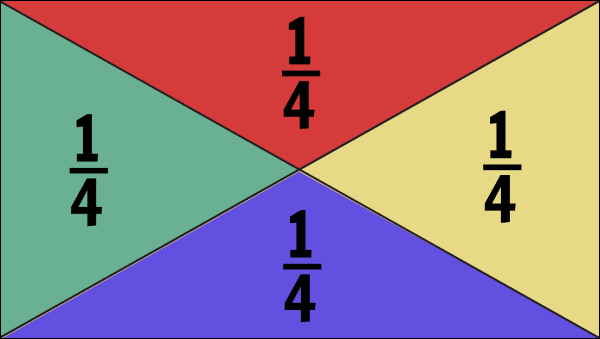Making the switch from whole numbers to parts and wholes can be very difficult for young minds. It is mind-boggling for some to learn that there are numbers between the counting numbers! Fractions is a topic with which many students struggle throughout elementary and middle school, so it is important that students have a thorough understanding of what fractions are, estimating and comparing amounts visually and numerically, and recognizing reasonable answers. The activities below aim to help students visualize and begin to master fractions and all that goes with them.

## Background Information

Many students confuse fractions with the whole numbers that they are so used to seeing. The number “1/3” looks like two different numbers rather than a single numerical value. A fraction is a number with an integer numerator and a nonzero denominator that, for our purposes, can represent rational numbers (1/4 or 3 2/5) and whole numbers (4/2 = 2). The “numerator” is the quantity in the top section of the fraction that represents the number of parts, and the “denominator” is the value below the fraction bar indicating the number of partitions or shares, known also as the “whole".

Fraction notation can indicate ratio and proportions, multiplicative relationships, quotient when dividing two numbers, measurement, and parts of wholes or sets. Beginning fraction masters need only worry about parts of wholes or sets and measurement, but astute students will likely notice multiplicative relationships (i.e. the half circle is twice the size of the quarter circle or, inversely, the quarter circle is 1/2 the size of the half circle) and dividing two numbers (sharing 7 cookies among 3 people would be written 7/3, the same as 7 ÷ 3).

### Student Prior Knowledge

Students should know that shapes can often be split into equal shares, such as halves, thirds, and quarters or fourths. The concept of sharing items, such as supplies or food, as well as sharing time fairly, like turn-taking or splitting the day into class periods/subjects, should be well established by this age. Draw on real-life examples whenever possible to strengthen understanding.

While it is not imperative, it is helpful if students are already familiar with multiplication and division. Mastery of basic facts is a separate skill from understanding and manipulating fractions, but understanding one may help the understanding of the other. Consider reviewing the multiplication/division facts as necessary.

Fun Fact: The fraction bar is called the vinculum!

This fraction lesson plan is a mini supplement on fractions to be used for remedial or extension work and information, teacher guidance and inspiration, alternative instruction, integrating writing and mathematics, or for whatever you wish!

Teachers can use Storyboard That to make short storyboards for a concept, discussion springboard, quick visual review before a unit or lesson, word problems, or as a slideshow presentation to accompany a lesson! Teachers can also create custom fraction worksheets to help students practice the concepts they find most difficult to master.

Students can use Storyboard That to write math stories, fraction word problems with realistic applications, explain a concept as an assessment, or explain a concept to show another student (student exchange).

Be sure to check out more of our Math resources!
*(This will start a 2-Week Free Trial - No Credit Card Needed)